# Stellar Heating

One of the sources of heat energy that can change the temperature of an object is heat received from stars in the simulation. This stellar energy is produced inside the star and radiated in all directions out into space. Any object with a direct line-of-sight to the star will receive a fraction of the energy.

## Models

Every star in a simulation radiates energy out into space. The rate at which the energy is emitted, also known as the emitted power, is called the star's Luminosity. For an object located a distance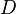$D$ from the star with luminosity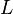$L$, the power per area received by the object is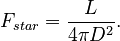$F_{star} = \frac{L}{4 \pi D^2}.$

Power per area is sometimes called flux density or irradiance.

The larger the object is, the more total power it will receive from the star. This total power depends on the cross-sectional area of the object. For a sphere (like a planet or moon) with radius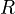$R$, cross-sectional area is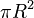$\pi R^2$, and the total power received from the star is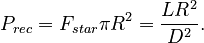$P_{rec} = F_{star} \pi R^2 = \frac{L R^2}{D^2}.$

A fraction of the received energy will be absorbed by the object, and the rest will be reflected back into space. Only the absorbed energy will affect the object's temperature. The fraction of energy reflected into space is represented by the object's albedo,$a$, so the rate at which energy is absorbed from the star, or the Stellar Power, is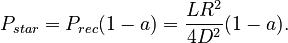$P_{star} = P_{rec}(1 - a) = \frac{L R^2}{4 D^2} (1 - a).$